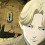## Tuesday, 11 August 2015

### Concurrent Quicksort Program in C using OPENMP

In this post, we will see how to implement Quick sort algorithm concurrently(parallelly) in C language. (For implementation in C++, check http://www.comrevo.com/2016/01/concurrent-quicksort-program-in-cpp-using-openmp.html). To make it concurrent/parallel, we will use OPENMP API. OPENMP is available alongwith gcc compiler.
In Quick sort, input is any sequence of numbers and output is Sorted array(here we will consider ascending order). We start with one number, mostly the first number, and finds its position in sorted array. This number is called pivot element. Then we divide the array into two parts considering this pivot element's position in sorted array. In each part, separately, we find the pivot elements. This process continues until all numbers are processed and we get the sorted array.
In Concurrent Quick sort, each part is processed by independent thread i.e. different threads will find out pivot element in each part recursively. Check in following diagram. Here PE stands for Pivot Element.

Array ----------------------------------------------------------------------------------

Array -----------------------------------------PE0-----------------------------------

Array -------------------PE1----------------PE0--------------PE2-----------------
.
.
.

Watch OpenMP Basics with Example in following video:

Program: (concurrentquicksort.c)

#include<stdio.h>
#include<omp.h>

int k=0;

int partition(int arr[], int low_index, int high_index)
{
int i, j, temp, key;
key = arr[low_index];
i= low_index + 1;
j= high_index;
while(1)
{
while(i < high_index && key >= arr[i])
i++;
while(key < arr[j])
j--;
if(i < j)
{
temp = arr[i];
arr[i] = arr[j];
arr[j] = temp;
}
else
{
temp= arr[low_index];
arr[low_index] = arr[j];
arr[j]= temp;
return(j);
}
}
}

void quicksort(int arr[], int low_index, int high_index)
{
int j;

if(low_index < high_index)
{
j = partition(arr, low_index, high_index);
printf("Pivot element with index %d has been found out by thread %d\n",j,k);

#pragma omp parallel sections
{
#pragma omp section
{
k=k+1;
quicksort(arr, low_index, j - 1);
}

#pragma omp section

{
k=k+1;
quicksort(arr, j + 1, high_index);
}

}
}
}

int main()
{
int arr;
int n,i;

printf("Enter the value of n\n");
scanf("%d",&n);
printf("Enter the %d number of elements \n",n);

for(i=0;i<n;i++)
{
scanf("%d",&arr[i]);
}

quicksort(arr, 0, n - 1);

printf("Elements of array after sorting \n");

for(i=0;i<n;i++)
{
printf("%d\t",arr[i]);
}

printf("\n");
}

How To Run:

To Compile:
>>>gcc -fopenmp concurrentquicksort.c
(Note: -fopenmp is used to add OPENMP libraries to the program)

To Run:
>>>./a.out

Output:

1.2.3.4.